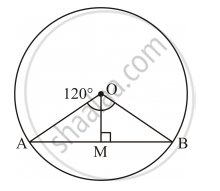# A Chord of a Circle of Radius 14 Cm Subtends an Angle of 120° at the Centre. Find the Area of the Corresponding Minor Segment of the Circle. [ U S E R π 22 7 and √ 3 = 1.73 ] - Mathematics

#### Question

A chord of a circle of radius 14 cm subtends an angle of 120° at the centre. Find the area of the corresponding minor segment of the circle. [User pi22/7 and sqrt3=1.73]

#### Solution

Let O be the centre of the circle and AB be the chord that subtends an angle of 120° at the centre.Here, OM ⊥ AB.

Radius of the circle, r = 14 cm

Area of the minor segment = Area of sector OAB − area of ΔAOB

text{Area of sector OAB}=O/ /360^@ xx pi r^2

=120^@/360^@xx22/7xx(14 cm)^2

=1/3xx22/7xx196 cm^2

=616/3 cm^2

Now ,OM ⊥ AB.

∴AM =MB =1/2AB [Perpendicular from the centre to the bisects the chord]

rArr AB =2AM

⇒ AB = 2AM

In ΔOAM and ΔOBM:

OA = OB [Radii of the same circle]

OM = OM [Common]

∠OMA = ∠OMB [Each 90°]

∴ ΔOAM ≅ ΔOBM [RHS congruence criterion]

⇒ ∠AOM = ∠BOM [C.P.C.T]

∴∠AOM =∠BOM= 120^@/2=60^@

In ΔAOD:

rArr sqrt3/2=(AM)/(OA), cos 60^@=(OM)/(OA)

rArr sqrt3/2=(AM)/(14 cm),1/2 = (OM)/(14cm)

∴ AB=2AM=14sqrt3cm

\text{Area} (Δ AOB)=1/2xxABxxOM=1/2xx14sqrt3xx7cm^2=49sqrt3cm^2

\text{Area of minor segment}=616/3 cm^2 -49 sqrt3 cm^2

=205.33cm^2-49xx1.73cm^2

=205.33cm^2-84.77 cm^2

=120.56 cm ^2

Thus, the area of the minor segment is 120.56 cm2.

Is there an error in this question or solution?

#### Video TutorialsVIEW ALL 

A Chord of a Circle of Radius 14 Cm Subtends an Angle of 120° at the Centre. Find the Area of the Corresponding Minor Segment of the Circle. [ U S E R π 22 7 and √ 3 = 1.73 ] Concept: Concept of Circle - Centre, Radius, Diameter, Arc, Sector, Chord, Segment, Semicircle, Circumference, Interior and Exterior..